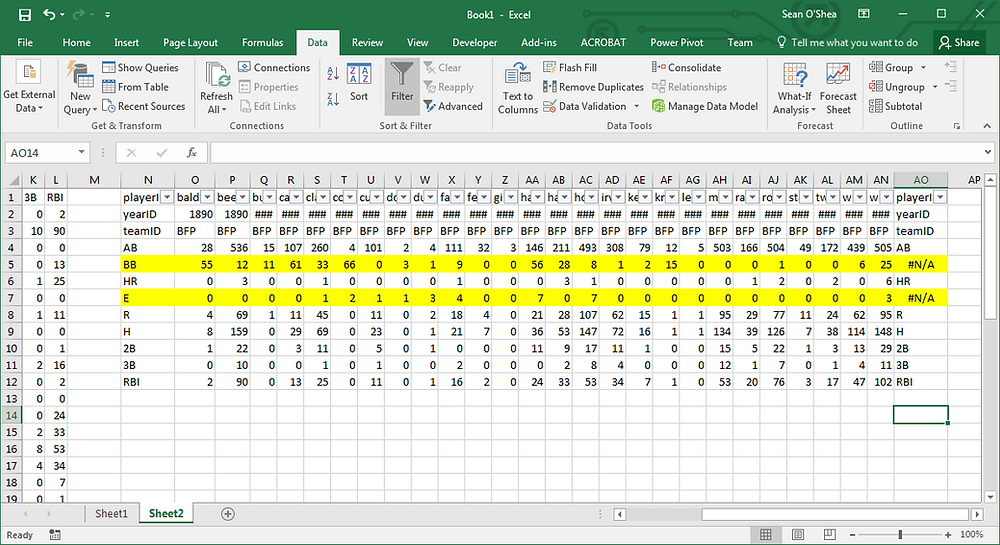Search

# Using HLOOKUP to merge data from two Excel files

HLOOKUP is the lesser known companion to VLOOKUP, the Excel workhorse formula. You can put HLOOKUP to good use when you have two Excel files with some but not all columns in common, and you want to merge them.

In this example we're using this formula:

=HLOOKUP(A\$1,Sheet2!\$1:\$100,ROW(A1),FALSE)

. . . to pull data from Sheet2 onto Sheet1. Sheet1 has the following headings:

playerID yearID stint teamID lgID G AB R H 2B 3B HR RBI SB

. . . and Sheet2 has these headings:

playerID yearID teamID AB BB HR E R H 2B 3B RBI

We want to add in the data from Sheet2 to the columns that are in common between the 2. So here:

=HLOOKUP(A\$1,Sheet2!\$1:\$100,ROW(A1),FALSE)

. . . refers to the first heading on Sheet1, and then searches the first 100 columns a match (\$1:\$100), and returns the entry from the first row (ROW(A1)). When this formula is pulled down and to the right it both collects the entries under the first heading from Sheet2 and also searches for the subsequent headings on the second worksheet.To find the columns from Sheet2 which are not used on Sheet1 you can use another HLOOKUP formula at the bottom of Sheet1:

=HLOOKUP(A1,Sheet1!1:1,1,FALSE)

. . . then simply transpose the data to filter it down to the columns missing from sheet1:. . . then re-transpose the data again so just the missing columns (in this example BB and E) are added to the first worksheet.